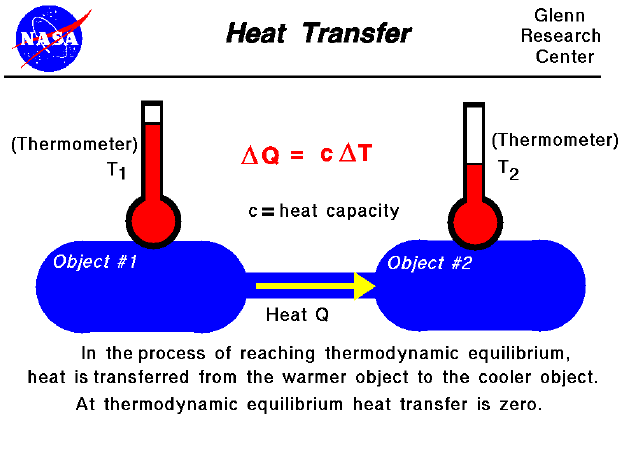Thermodynamics is a branch of physics that deals with the energy and work of a system. As mentioned on the gas properties slide, thermodynamics deals only with the large scale response of a system that we can observe and measure in experiments. As aerodynamicists, we are most interested in the thermodynamics of propulsion systems.

The Zeroth Law of Thermodynamics introduces the concept of thermodynamic equilibrium, in which two objects have the same temperature. If we bring two objects that are initially at different temperatures into physical contact, they will eventually achieve thermal equilibrium. During the process of reaching thermal equilibrium, heat is transferred between the objects. The amount of heat transferred is proportional to the temperature difference between the objects and the heat capacity of the object. The heat capacity is a constant that tells how much heat is added per unit temperature rise. The value of the constant is different for different materials. Heat is always transferred from the object at the higher temperature to the object with the lower temperature.

For a gas, the heat transfer is related to a change in temperature. The temperature, pressure, and volume of the gas determine the state of the gas. Heating a gas changes the state of the gas. But the state of a gas can be changed in a wide variety of ways. On another slide, we show how work done on a gas also changes the state of the gas. The amount of work that a gas can do depends on both the initial and final states and on the process used to make the change. In the same way, the amount of heat transferred in changing the state of a gas also depends on the initial and final states and the exact process used to change the state. Different processes result in different amounts of heat transfer and work. The effects of both heat flow and work are combined in the First Law of Thermodynamics.

Guided Tours